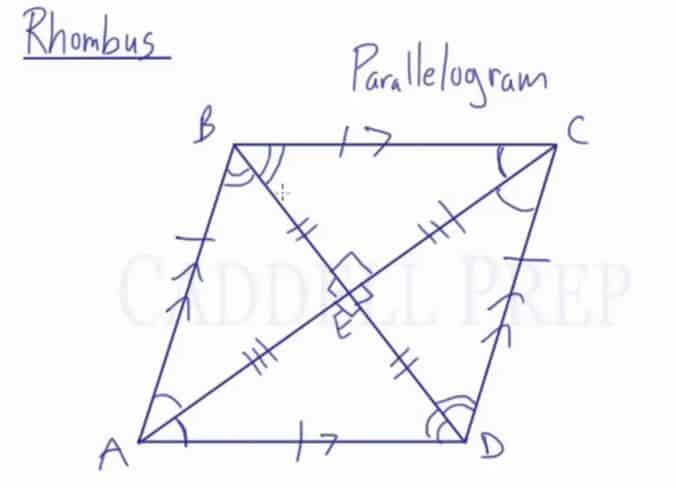Watch a video lesson and learn about the properties of rhombuses including properties of sides, angles, diagonals and angles formed by diagonals.

Rhombus: A parallelogram with consecutive sides congruent.

A rhombus has a similar set of rules as a parallelogram does.

The special thing about a rhombus is that all 4 sides are congruent.

The diagonals are perpendicular bisectors to each other. When this happens, four congruent triangles are formed. The diagonals also bisect the angles at each vertex.## Video-Lesson Transcript

Let’s go over a rhombus.

A rhombus is another type of parallelogram.

In a parallelogram, we have opposite sides are parallel. And also opposite sides are congruent.

What’s special about a rhombus is that all four sides are congruent. Not only the opposite sides but all four of them are congruent.

Let’s label these vertices.

When we draw diagonals, they bisect each other.

Something else that happens in a rhombus is when diagonals intersect, we get right angles. The diagonals are perpendicular to each other.

Here, we end up with four congruent right triangles.

This diagonal bisects$\angle A$. So, angle$BAD$ are bisected. Angle$BCD$ also gets bisected.

And also, the second diagonal bisects these two angles. Angle$ABC$ and angle$ADC$ such that these two pairs of angles are congruent.